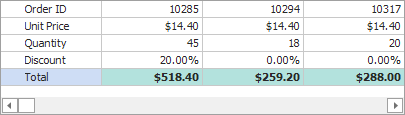# RowProperties.UnboundDataType Property

Allows you to make the row unbound, and specify the type of data it stores.

Namespace: DevExpress.XtraVerticalGrid.Rows

Assembly: DevExpress.XtraVerticalGrid.v22.2.dll

NuGet Package: DevExpress.Win.VerticalGrid

## Declaration

``````[DXCategory("Data")]
public Type UnboundDataType { get; set; }``````

## Property Value

Type Description
Type

The type of data to store in the unbound row.

## Remarks

If the UnboundDataType property is not set to a valid data type (the property’s default value is System.Void), the current row is considered bound to a data source field (RowProperties.FieldName).

To switch a row to unbound mode, do the following:

• Set the UnboundDataType property to the type of values this row should store.
• Assign a unique field name to the RowProperties.FieldName property. The field name must not match the field name of another row nor the name of a data source field.
• Use one of the following techniques to populate the unbound row with data:

## Example

In the following example, it is assumed that the Vertical Grid is bound to a table that contains the “Quantity”, “UnitPrice” and “Discount” fields. The code below adds an unbound row that calculates the total order amount according to the following expression: Quantity*UnitPrice*(1-Discount).``````using DevExpress.XtraVerticalGrid;
using DevExpress.XtraVerticalGrid.Rows;
using DevExpress.Data;
using DevExpress.Utils;
using DevExpress.XtraVerticalGrid.Events;

// Unbound row.
EditorRow rowTotal = null;

private void Form1_Load(object sender, EventArgs e) {
// ...
vGridControl1.CustomUnboundData += new CustomDataEventHandler(vGridControl1_CustomUnboundData);
// Create and initialize the unbound Total row.
rowTotal = new EditorRow();
rowTotal.Properties.Caption = "Total";
rowTotal.Properties.FieldName = "Total";
// Specify format settings.
rowTotal.Properties.Format.FormatType = FormatType.Numeric;
rowTotal.Properties.Format.FormatString = "c";
// Disable edit operations.
rowTotal.Properties.UnboundDataType = typeof(decimal);
// Customize the appearance settings.
rowTotal.Appearance.BackColor = Color.FromArgb(179, 226, 221);
rowTotal.Appearance.Font = new System.Drawing.Font(rowTotal.Appearance.Font,
System.Drawing.FontStyle.Bold);
}

// Provide data for the Total row.
private void vGridControl1_CustomUnboundData(object sender, CustomDataEventArgs e) {
VGridControl vGrid = sender as VGridControl;
if (e.Row == rowTotal && e.IsGetData) {
DataRowView row = (DataRowView)vGrid.GetRecordObject(e.ListSourceRowIndex);
decimal unitPrice = Convert.ToDecimal(row["UnitPrice"]);
decimal quantity = Convert.ToDecimal(row["Quantity"]);
decimal discount = Convert.ToDecimal(row["Discount"]); ;
e.Value = unitPrice * quantity * (1 - discount);
}
}
``````